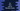# 4 ways in C# to find the sum of first n numbers of the Fibonacci series## How to find the sum of first n numbers of the Fibonacci series in C#:

In this post, we will learn how to find the sum of first n numbers of the Fibonacci series in C#. The program will take the value of n as the input and print the sum.

The Fibonacci series starts with 0 and 1 and each value in the series is equal to the sum of previous two numbers of the series. The first 10 numbers of the series are 0, 1, 1, 2, 3, 5, 8, 13, 21, 34.

### Method 1: C# program to find the sum of first n numbers of the Fibonacci series by using a for loop:

In this method, we will use a for loop to find the sum of the first n numbers of the Fibonacci series.

``````using System;

namespace Program
{
class Program
{
static void Main(string[] args)
{
int n;
int CurrentValue = 1, PrevValue = 0, Sum = 1, CurrentSum;

Console.WriteLine("Enter the value of n: ");

if(n == 0) Sum = 0;

for (int i = 2; i < n; i++)
{
CurrentSum = PrevValue + CurrentValue;
PrevValue = CurrentValue;
CurrentValue = CurrentSum;
Sum += CurrentValue;
}

Console.WriteLine("Sum of first {0} numbers of the Fibonacci series is: {1}", n, Sum);
}
}
}``````

In this example,

• The for loop is used to print the sum.
• PrevValue and CurrentValue are integer variables to hold the previous and current values of the series. These are assigned as 0 and 1 at the start of the program.
• Inside the loop, it finds the final Sum. On each iteration, it calculates the sum of the previous two values of the series to find the current value. This value is added to Sum.

If you run this program, it will print output as like below:

``````Enter the value of n:
6
Sum of first 6 numbers of the Fibonacci series is: 12``````

### Method 2: By using a separate method:

Let’s use a separate method to find the sum of first n numbers of the Fibonacci series:

``````using System;

namespace Program
{
class Program
{
static int FindSumNFibonacci(int n)
{
int CurrentValue = 1, PrevValue = 0, Sum = 1, CurrentSum;

if (n == 0) Sum = 0;

for (int i = 2; i < n; i++)
{
CurrentSum = PrevValue + CurrentValue;
PrevValue = CurrentValue;
CurrentValue = CurrentSum;
Sum += CurrentValue;
}

return Sum;
}
static void Main(string[] args)
{
int n;

Console.WriteLine("Enter the value of n: ");

Console.WriteLine("Sum of first {0} numbers of the Fibonacci series is: {1}", n, FindSumNFibonacci(n));
}
}
}``````
• FindSumNFibonacci is a different method to find the sum of first n numbers of the Fibonacci series. It takes the value of n as its parameter and returns the sum.
• This is similar to the above program. It uses the for loop to calculate the sum. The variable Sum holds the sum.
• At the end of the program, it returns the sum, i.e. the value of Sum.

It will give similar result.

``````Enter the value of n:
7
Sum of first 7 numbers of the Fibonacci series is: 20``````

### Method 3: By using a while loop:

Let’s replace the for loop with a while loop:

``````using System;

namespace Program
{
class Program
{
static int FindSumNFibonacci(int n)
{
int CurrentValue = 1, PrevValue = 0, Sum = 1, CurrentSum, i = 2;

if (n == 0) Sum = 0;

while (i < n)
{
CurrentSum = PrevValue + CurrentValue;
PrevValue = CurrentValue;
CurrentValue = CurrentSum;
Sum += CurrentValue;
i++;
}

return Sum;
}
static void Main(string[] args)
{
int n;

Console.WriteLine("Enter the value of n: ");

Console.WriteLine("Sum of first {0} numbers of the Fibonacci series is: {1}", n, FindSumNFibonacci(n));
}
}
}``````

The only difference is that the value of i is initialized at the start of the method and it’s value is incremented at the end of each iteration of the loop. You will get similar result.

### Method 4: Recursive method to find the sum of first n numbers of the Fibonacci series:

A recursive method calls itself again and again to find a result. We can use a recursive method to find the sum of first n numbers of a Fibonacci series. Let’s write down the program:

``````using System;

namespace Program
{
class Program
{
static int GetSumNFibonacciSeries(int First, int Second, int n, int Sum)
{
if (n == 0) return Sum;

return GetSumNFibonacciSeries(Second, First + Second, n - 1, Sum + First);
}
static void Main(string[] args)
{
int n;

Console.WriteLine("Enter the value of n: ");

Console.WriteLine("Sum of first {0} numbers of the Fibonacci series is: {1}", n, GetSumNFibonacciSeries(0, 1, n, 0));
}
}
}``````
• GetSumNFibonacciSeries method is used to find the sum of first n numbers of the Fibonacci series.
• It takes 4 parameters. First is the first value of the series, Second is the second value of the series in the beginning. These values are updated on each recursive call. n is the value of n entered by the user. Sum is to hold the final sum. It is 0 in the beginning.
• On each iteration, it calls itself recursively with updated First, Second, n and Sum values.
• The value of n is decremented by 1 on each recursive call. It returns the value of Sum once the value of n become 0.

It will give similar result.

``````Enter the value of n:
8
Sum of first 8 numbers of the Fibonacci series is: 33

Enter the value of n:
9
Sum of first 9 numbers of the Fibonacci series is: 54``````